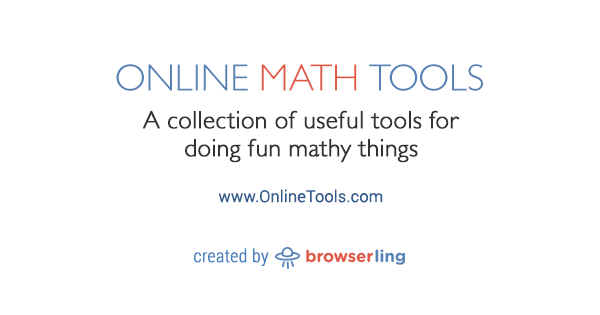Today we're releasing the thirteenth site.

The thirteenth site in our network is Online Math Tools. Online Math Tools is a collection of simple, free and easy to use utilities for doing all kinds of mathy things. There are no ads, popups or other garbage. Just math utilities that work in your browser. Load variables and instantly get result.www.onlineMATHtools.com

Here's a list of all math tools:

Here are upcoming math tools:

• Find n-th e Digit
• Find n-th Golden Ratio Digit
• Find n-th Pi Digit
• Find Prime Factors
• Test if a Number is a Prime
• Test if a Number is a Fibonacci Number
• Decode a Look-and-say Sequence
• Generate a Moser-de Bruijn Sequence
• Generate a Random Matrix
• Generate an Identity Matrix
• Check if a Matrix is Singular
• Find the Co-factor Matrix
• LU Factor a Matrix
• Find Matrix Eigenvalues
• Draw a Mandelbrot Fractal
• Draw a Julia Fractal
• Draw a Rauzy Fractal
• Draw a Vicsek Fractal
• Draw a Quadric Cross Fractal
• Draw a T-square Fractal
• Draw a Sierpinski Carpet Fractal
• Draw a Sierpinski Pentagon Fractal
• Draw a Sierpinski Hexagon Fractal
• Draw a Fibonacci Word Fractal
• Draw Cantor Set Fractal
• Draw Asymmetric Cantor Set Fractal
• Draw Generalized Cantor Set Fractal
• Draw Smith-Volterra-Cantor Set Fractal
• Draw Cantor Dust Fractal
• Draw Blancmange Fractal Curve
• Draw Weierstrass Function
• Draw Minkowski Question-mark Curve
• Draw Thomae's Function
• Draw Dirichlet's Function

The next few sites are onlineIMAGEtools, onlineUTF8tools, onlineGIFtools, onlineBMPtools, onlinePDFtools, onlineHASHtools, onlineBROWSERtools, onlineCRYPTOtools, onlineAUDIOtools, onlineCSStools, onlineJStools, and then 10 more.

If you like what I'm doing you can subscribe to my blog and/or follow me on twitter and/or follow browserling on twitter and/or do nothing.

See you next time!